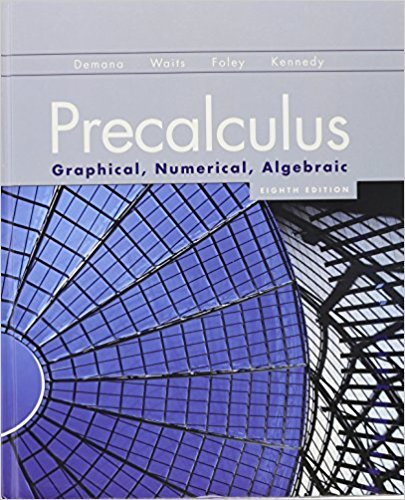×
×

# Solutions for Chapter 1.7: Functions and Graphs## Full solutions for Precalculus: Graphical, Numerical, Algebraic | 8th Edition

ISBN: 9780321656933Solutions for Chapter 1.7: Functions and Graphs

Solutions for Chapter 1.7
4 5 0 373 Reviews
23
0
##### ISBN: 9780321656933

Chapter 1.7: Functions and Graphs includes 62 full step-by-step solutions. Since 62 problems in chapter 1.7: Functions and Graphs have been answered, more than 45634 students have viewed full step-by-step solutions from this chapter. This textbook survival guide was created for the textbook: Precalculus: Graphical, Numerical, Algebraic, edition: 8th Edition. Precalculus: Graphical, Numerical, Algebraic was written by and is associated to the ISBN: 9780321656933. This expansive textbook survival guide covers the following chapters and their solutions.

Key Calculus Terms and definitions covered in this textbook
• Anchor

See Mathematical induction.

• Coefficient of determination

The number r2 or R2 that measures how well a regression curve fits the data

• Compound interest

Interest that becomes part of the investment

• Conjugate axis of a hyperbola

The line segment of length 2b that is perpendicular to the focal axis and has the center of the hyperbola as its midpoint

• Difference of two vectors

<u1, u2> - <v1, v2> = <u1 - v1, u2 - v2> or <u1, u2, u3> - <v1, v2, v3> = <u1 - v1, u2 - v2, u3 - v3>

• Distributive property

a(b + c) = ab + ac and related properties

• Inductive step

See Mathematical induction.

• Instantaneous velocity

The instantaneous rate of change of a position function with respect to time, p. 737.

• Inverse cosecant function

The function y = csc-1 x

• Irrational numbers

Real numbers that are not rational, p. 2.

• Length of an arrow

See Magnitude of an arrow.

• Linear inequality in x

An inequality that can be written in the form ax + b < 0 ,ax + b … 0 , ax + b > 0, or ax + b Ú 0, where a and b are real numbers and a Z 0

• Order of magnitude (of n)

log n.

• Orthogonal vectors

Two vectors u and v with u x v = 0.

• Perpendicular lines

Two lines that are at right angles to each other

The formula x = -b 2b2 - 4ac2a used to solve ax 2 + bx + c = 0.

A function that can be written in the form ƒ(x) = ax 2 + bx + c, where a, b, and c are real numbers, and a ? 0.

• Riemann sum

A sum where the interval is divided into n subintervals of equal length and is in the ith subinterval.

• Sum of complex numbers

(a + bi) + (c + di) = (a + c) + (b + d)i

• Synthetic division

A procedure used to divide a polynomial by a linear factor, x - a

×